# ISEE Upper Level Math : How to find the length of the side of other quadrilaterals

## Example Questions

### Example Question #1 : Quadrilaterals

The perimeter of a quadrilateral is 86. The four sides measure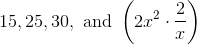.

What is the value of?Explanation:

Given that the perimeter is 86, the sides of the quadrilateral will all add up to this amount.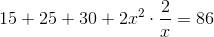The first step is the add together all the integers.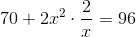Next, we subtract 70 from each side.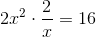We reduce the left side of the equation: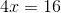Now, we divide each side by 4. This leaves: